July 17, 2019

# Introduction

• B-trees are balanced search trees
• Designed to work on secondary storage devices (i.e. disks)
• Similar to red-black trees
• Better minimizing disk I/O operations
• Database systems use B-trees (or variants)

# Introduction

• Reminder on Red-black trees (brief…)
• A red-black tree is a binary tree, satisfies the red-black properties
1. Every node is either red or black
2. The root is black
3. Every leaf (NIL) is black
4. If a node is red, then both its children are black
5. For each node: all simple paths from node to descendant leaves contain the same number of black nodes
• Black height of a node
• The number of black nodes on any simple path from, but not including, a node $$x$$ down to a leaf
• $$bh(x)$$
• Red black trees make good search trees
• Lemma 13.1, “A red-black tree with $$n$$ internal nodes has height at most $$2 \lg(n+1)$$
• Operations
• Rotation, $$O(1)$$
• Insertion, $$O(\lg n)$$
• Deletion, $$O(\lg n)$$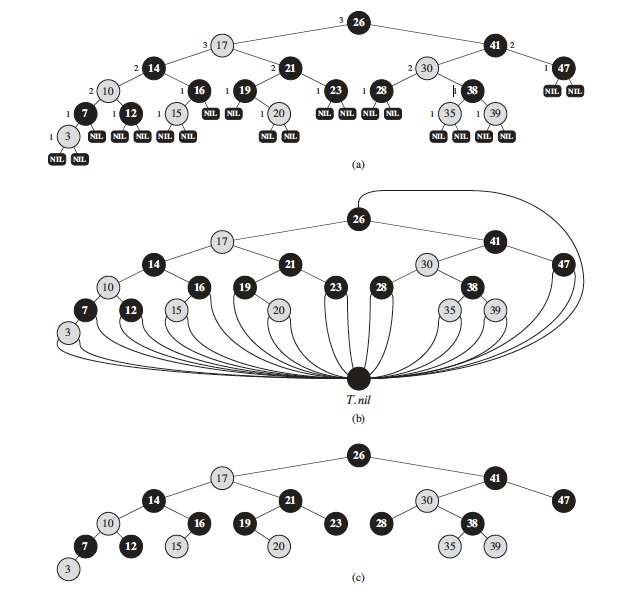# Introduction

• When do we use a B-tree?
1. Keeps records in sorted order for sequential traversing
2. Uses a hierarchical index to minimize number of disk reads
3. Uses partially-full blocks to speed insertions and deletions
4. Index is elegantly adjusted with recursive algorithm
• Additional constraints, to ensure the tree is always balanced

# Introduction

• B-trees vs red-black trees
• B-tree nodes may have many children (from a few to thousands)
• Branching factor can be large
• Depends on characteristics of the disk unit
• Every $$n$$-node B-tree has height $$O(\lg n)$$, as in red-black trees
• Height of B-tree can be much less than that of a red-black tree
• Because of its branching factor
• Base of logarithm expressing its height can be much larger
• Can use B-trees to implement many dynamic-set operations in time $$O(\lg n)$$

# Introduction

• B-trees generalize binary search trees in natural manner
• If internal node $$x$$ contains $$x.n$$ keys, then $$x$$ has $$x.n+1$$ children
• Keys in node $$x$$ are dividing points separating the range of keys handled by $$x$$ into $$x.n +1$$ subranges
• Each subrange handled by a child of $$x$$
• When searching for a key
• We make an $$(x.n + 1)$$-way decision based on comparisons with the $$x.n$$ keys stored in $$x$$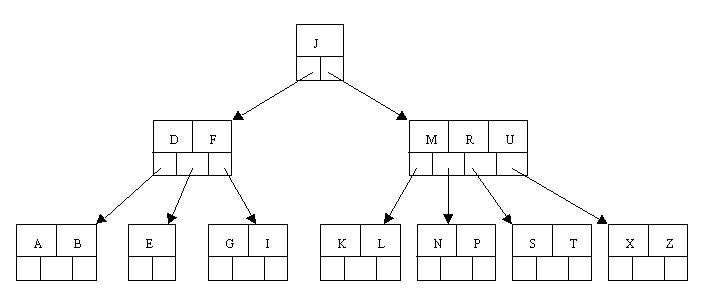# Introduction

• A b-tree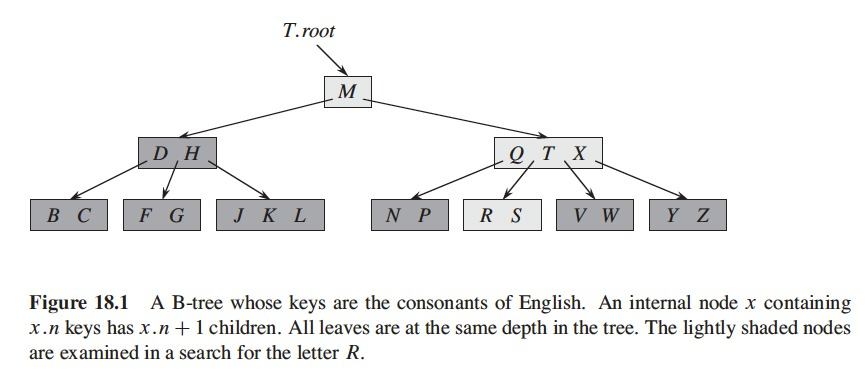# Data Structures on Secondary Storage

• Computer systems use technology for memory capacity
• Main memory
• Silicon memory chips
• More expensive per bit stored than magnetic storage technology (tapes or disks)
• Secondary storage
• Based on magnetic disks
• Amount of this storage often exceeds the amount of primary memory (by two or more orders of magnitude)

# Data Structures on Secondary Storage

• Typical disk drive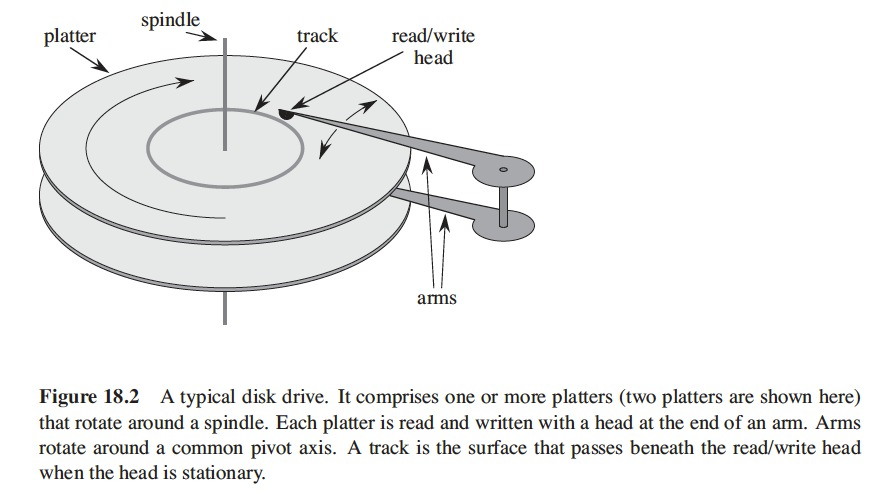# Data Structures on Secondary Storage

• Disks are cheaper than memory but they are much, much slower
• Because they move mechanical parts
• Platter rotation
• Arm movement
• Disks rotate at speeds of 5,400 - 15,000 RPM
• 1 rotation takes 8.33 milliseconds (5 orders of magnitude longer than silicon memory)
• Silicon memory access takes about 50 nanoseconds
• In a full rotation we could access main memory more than 100,000 times

# Data Structures on Secondary Storage

• Amortize the time spent waiting for mechanical movements
• Disk access more than 1 item at a time
• Information divided into equal-sized pages of bits
• Appear consecutively within tracks
• A disk read or write is of one or more entire pages
• Typical disk page is $$2^{11}$$ to $$2^{14}$$ bytes long
• Reading or writing is entirely electronic (aside from rotation of disk)
• Disk can read or write large amounts of data quickly
• Often, accessing a page of information and reading it from disk takes longer than examining all information read
• Look separately two principal components of the running time
• The number of disk accesses
• In terms of number of pages of information (read/written to disk)
• The CPU (computing) time

# Data Structures on Secondary Storage

• A typical B-tree application
• Amount of data too large
• Not all data fit into memory
• B-tree algorithms copy selected pages from disk to memory
• As needed
• Write back into disk pages that change
• Keep only constant number of pages in memory (at any time)
• Size of memory doesn’t limit size of B-trees that can be handled

# Data Structures on Secondary Storage

• Disk operations
• Let $$x$$ be a pointer to an object
• If object already in main memory
• $$x.key$$
• If object in disk
• DISK-READ($$x$$), to read object into main memory, if object already in memory, no DISK-READ operation performed
• DISK-WRITE($$x$$), to save changes made to attributes of object $$x$$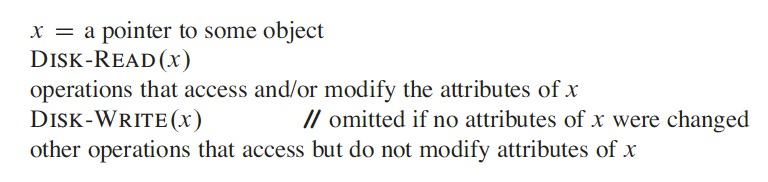# Data Structures on Secondary Storage

• Because system keeps limited number of pages in main memory at any time
• System flushes from memory pages no longer in use
• Our B-tree algorithm ignores this fact
• B-tree node is usually as large as a whole disk page
• In order to read/write as much information as possible, as a whole disk page
• This size limits the number of children a B-tree node can have
• Large B-tree stored on a disk
• Branching factors from 50 to 2,000
• Depending on size of key relative to size of a page
• Large branching factor
• Reduces height of tree and number of disk accesses to find any key

# Data Structures on Secondary Storage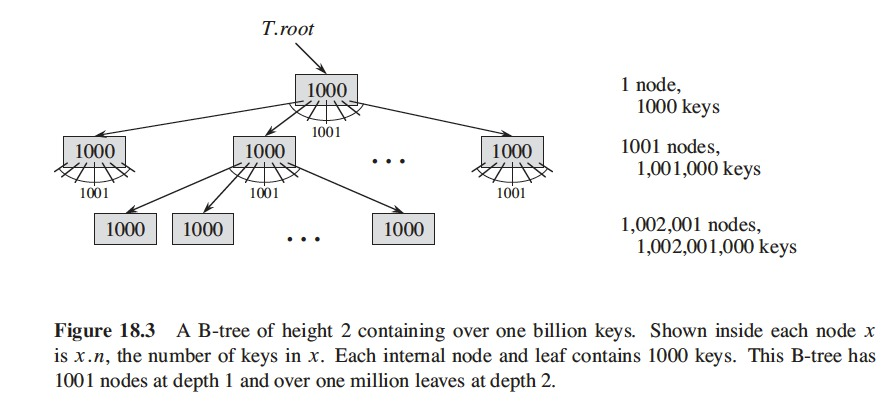# Definition of B-trees

• Assume that satellite information associated with a key resides in the same node as the key
• $$B^{+}-tree$$ are different, they store satellite information in leaves and stores only keys and child pointers in internal nodes
• Maximize branching factor of internal nodes

# Definition of B-trees

• A B-tree $$T$$ is a rooted tree (with root $$T.root$$) with the following properties
1. Every node $$x$$ has the following attributes:
1. $$x.n$$, the number of keys currently stored in node $$x$$
2. the $$x.n$$ keys themselves, $$x.key_1 \leq x.key_2, \dots, x.key_{x.n}$$, stored in nondecreasing order, so that $$x.key_1 \leq x.key_2 \dots \leq x.key_{x.n}$$,
3. $$x.leaf$$, a boolean value that is TRUE if $$x$$ is a leaf and FALSE if $$x$$ is an internal node
2. Each internal node $$x$$ also contains $$x.n + 1$$ pointers $$x.c_1, x.c_2, \dots, x.c_{x.n+1}$$ to its children. Leaf nodes have no children ($$c_i$$ attributes undefined)
3. The keys $$x.key_i$$ separate the ranges of keys stored in each subree: if $$k_i$$ is any key stored in the subtree with root $$x.c_i$$, then
• $$k_1 \leq x.key_1 \leq k_2 \leq x.key_2 \leq \dots \leq x.key_{x.n} \leq k_{x.n+1}$$.
4. All leaves have the same depth, which is the tree’s height $$h$$.
5. Nodes have lower and upper bounds on the number of keys they can contain. We express these bounds in terms of a fixed integer $$t \geq 2$$ called the minimum degree of the B-tree
1. Every node other than the root must have at least $$t-1$$ keys.
• Internal nodes at least $$t$$ children.
• If tree is nonempty, the root must have at least one key.
2. Every node may contain at most $$2t-1$$ keys.
• An internal node may have at most $$2t$$ children
• A node is full if it contains exactly $$2t-1$$ keys.
• Simplest B-tree
• When $$t = 2$$
• Internal nodes have 2, 3, or 4 children
• A 2-3-4 tree

# The Height of a B-tree

• Number of disk accesses in a B-tree is proportional to the height of the B-tree
• Worst-case height of a B-tree!
• Theorem 18.1
• If $$n \geq 1$$, then for any $$n$$-key B-tree $$T$$ of height $$h$$ and minimum degree $$t \geq 2$$, $$h \leq \log_t \frac{n+1}{2}$$.
• Proof
• The root of a B-tree $$T$$ contains at least one key
• All other nodes contain at least $$t-1$$ keys
• $$T$$ with height $$h$$ has at least 2 nodes at depth 1
• At least $$2t$$ nodes at depth 2
• At least $$2t^2$$ at depth 3, and so on
• At depth $$h$$ it has at least $$2t^{h-1}$$ nodes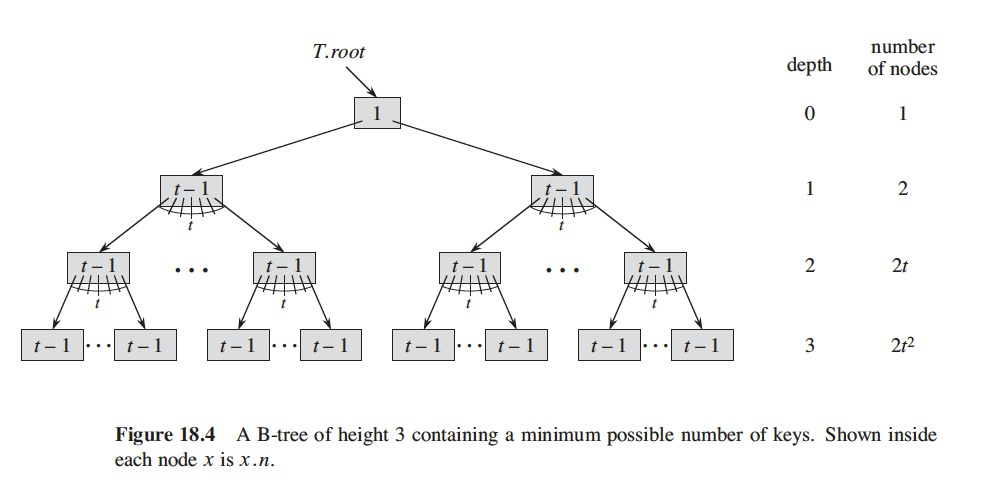• The number $$n$$ of keys satisfies the inequality
• $$n \geq 1 + (t-1) \sum\limits_{i=1}^{h}2t^{i-1}$$
• $$= 1 + 2(t-1) ( \frac{t^h - 1}{t - 1} )$$
• $$=2t^h - 1$$
• We get $$t^h \leq (n+1)/2$$ (by simple algebra)
• We take base-$$t$$ logarithms to both sides and prove the theorem.
• B-trees are powerful
• Save factor of about $$\lg t$$ over red-black trees
• In number of nodes examined for most tree operations
• Avoid substantial number of disk accesses

# Basic Operations on B-trees

• Conventions
• The root of the B-tree is always in main memory
• No DISK-READ on the root
• We do DISK-WRITE when root node changes
• Procedures are all “one-pass” algorithms
• Proceed downward from the root
• Don’t back up

# Basic Operations on B-trees

• Searching a B-tree
• Similar to searching a binary search tree
• Instead of making a binary branching decision at each node
• We make a multiway branching decision
• According to the node’s children
• An ($$x.n+1$$)-way branching decision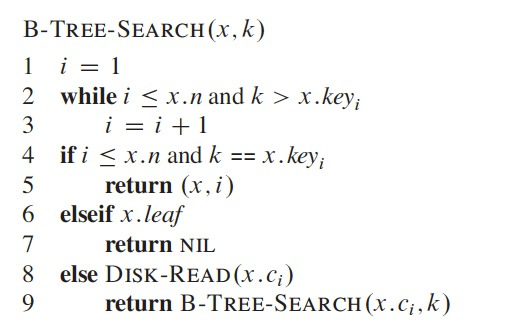• B-TREE-SEARCH accesses $$O(h) = O(\log_t n)$$ disk pages
• $$h$$ is the height of the B-tree
• $$n$$ is the number of keys in the B-tree
• Because $$x.n < 2t$$
• While loop (lines 2-3) takes $$O(t)$$ time in each node
• Total CPU time is $$O(th) = O(t \log_t n)$$.

# Basic Operations on B-trees

• Creating an empty B-tree
• We create an empty root node
• Then we can insert keys into that tree
• Require the ALLOCATE-NODE procedure
• Allocates a disk page to be used as a new node, $$O(1)$$ time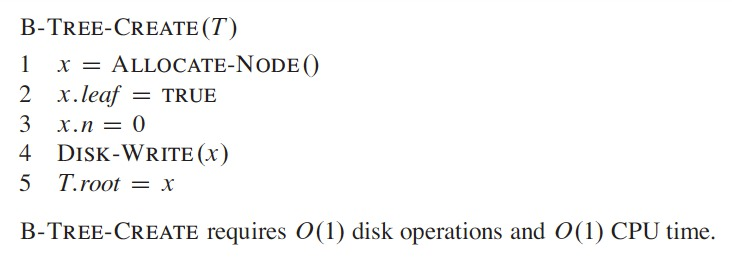# Basic Operations on B-trees

• Inserting a key into a B-tree
• More complicated than inserting a key in a binary tree
• Search for the leaf position to insert the new key
• Can’t simply create new leaf node and insert it, would fail to have a valid B-tree
• We insert the new key into an existing leaf node
• We cannot insert if node is full
• Introduce new operation that splits a full node
• Full node $$y$$ has $$2t-1$$ keys
• Split around the median key $$y.key_t$$ into two nodes with only $$t-1$$ keys each
• Median key moves up into $$y$$’s parent, but if $$y$$’s parent is full, we must split it… and so on
• We insert in a single pass down the tree from the root to a leaf
• Don’t wait to find out if we need to split a full node
• As we travel down the tree, we split each full node we find (including the leaf)
• So, when we split a full node $$y$$, we know its parent is not full

# Basic Operations on B-trees

• Splitting a Node in a B-tree
• B-TREE-SPLIT-CHILD
• Input: nonfull internal node $$x$$ and an index $$i$$ such that $$x.c_i$$ is a full child of $$x$$
• Splits this child in two and adjusts $$x$$ to have an additional child
• To split the root
• First make the root a child of a new empty root node
• Then we can use B-TREE-SPLIT-CHILD
• The tree only grows through splitting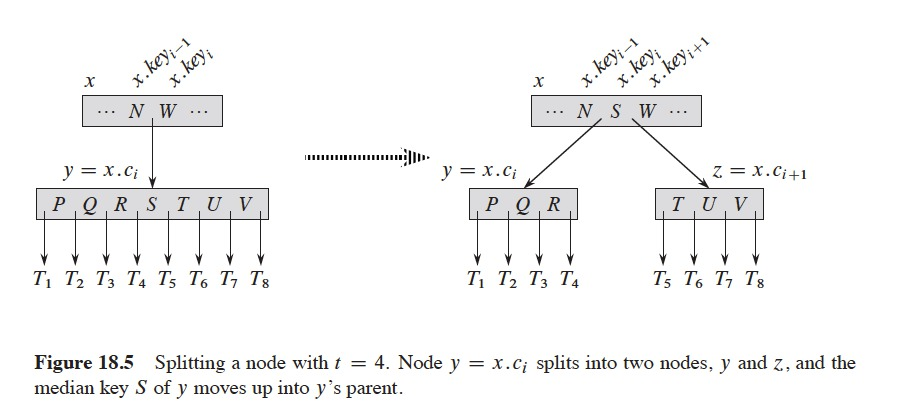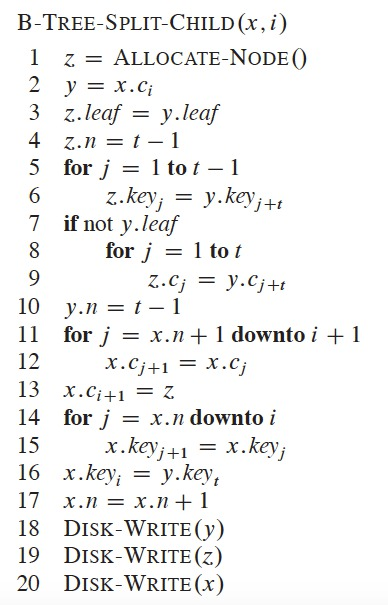• B-TREE-SPLIT-CHILD works as follows
• $$x$$ is the parent of the node being split
• $$y$$ is $$x$$’s $$i^{th}$$ child (the node being split)
• Node $$y$$ had originally $$2t$$ children and $$2t-1$$ keys
• Was reduced to $$t$$ children and $$t-1$$ keys
• Node $$z$$ takes the $$t$$ largest children, $$t-1$$ keys, from $$y$$
• $$z$$ becomes a new child of $$x$$
• Positioned after $$y$$ in $$x$$’s table of children
• Median key of $$y$$ moves up and becomes a key in $$x$$, it separates $$y$$ and $$z$$

# Basic Operations on B-trees

• Inserting a Key into a B-tree in a Single Pass Down the Tree
• A single pass down the tree
• $$O(h)$$ disk accesses
• CPU required is $$O(th) = O(t \log_t n)$$
• Uses B-TREE-SPLIT-CHILD
• Guarantee recursion never descends to a full node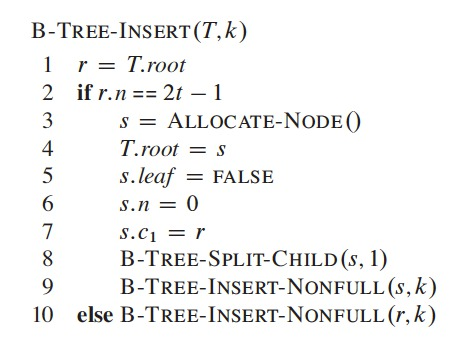• Lines 3-9, case in which root node $$r$$ is full
• Root splits and new node $$s$$ becomes the root
• Splitting the root is the only way to increase the height of a B-tree
• Increases height at the top, instead of at the bottom as happens in a binary search tree